### Home > MC1 > Chapter 5 > Lesson 5.2.2 > Problem5-55

5-55.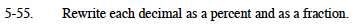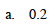The decimal 0.3 is equal to 30% and

$\frac{3}{10}$

$\text{0.2 is equal to 20\% and \frac{2}{10} \;or \frac{1}{5}}$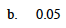Refer to part (a). Remember that this represents five hundredths.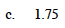The decimal 2.25 is equal to 225%.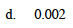If you divide part (a) by 100, the resulting decimal is 0.002. Try dividing your answers for part (a) by 100 to solve this problem.

$0.002\;=\;0.2\%\;=\;\frac{2}{1000}\;=\;\frac{1}{500}$